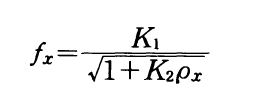# Pipe vibration density meters

## Density:

Density is a basic variable in the determination of the nature or composition of a substance. The standard units for measuring density are kg/m3 or g/cm3.

## Pipe vibration density meter:

A vibration-type density meters, which use change in the vibrational frequency of a pipe; and radiation density meters, which use change in the transmittance of radiation. Of these, we will explain the widely used vibration density meter and radiation density meter. If fx is the frequency of free vibration in the horizontal direction of a pipe of uniform cross section filled with liquid, the following equation is given:Here, Px is the density of the liquid being measured, K1 and Kz are constants determined by the inside and outside diameters of the pipe, the pipe material, the modulus of longitudinal elasticity, and the length of the vibrating part of the pipe.

Two pipes are used. These are symmetrical in the direction of vibration, so forces applied to the upper and lower connectors negate each other so no energy escapes to the outside (the main pipe system), and Q becomes large. The pipe walls are made 0.15 mm thick, increasing sensitivity. The vibrator becomes a kind of tuning fork. Combined with a positive feedback self- exciting oscillator circuit as oscillating element, this vibrator vibrates continuously.